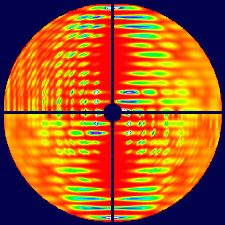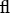# issue contentsFOUNDATIONSADVANCES
ISSN: 2053-2733

# March 1999 issueCover illustration: One quadrant of each of four fibre diffraction patterns from disordered polynucleotide fibres. The patterns were calculated for fibres with disorder as follows. Upper left: crystalline (no disorder); upper right: noncrystalline (completely disordered); lower left: discrete rotational disorder and correlated lattice disorder; lower right: random screw disorder. See Stroud & Millane [Acta Cryst. (1996), A52, 812-829].

## research papers

### Application of the statistical dynamical theory to-ray diffraction experiments on low-dislocation quartz single crystals

The experimental verification of the statistical dynamical theory of diffraction has been carried out by means of short-wavelength highly monochromatic γ-ray diffractometry. Dislocation-free silicon and low-dislocation quartz single crystals have been used as the experimental objects.

### X-ray standing wave as a result of only the imaginary part of the atomic scattering factor

A study of the forms of X-ray standing waves (XSW) when the real part of the scattering factor is zero shows that the effect of the anomalous transmission and absorption are connected with the phase of the XSW.

### Geometrical effects in diffraction analysis

The role of partial beam coherence and its interplay with finite scattering volumes in diffraction experiments is discussed. Under certain conditions, the analysis of diffraction profiles in terms of a sample response function convolved with an instrumental resolution function may fail and, further, under high absorption, new information of the source of scattering below the sample surface may become available.

### Elastic scattering of partially coherent beams of fast electrons by a crystal with a defect

Scattering of a quasi-monochromatic electron beam by a crystal with a defect is described with the use of the mutual coherency function and the formalism of quasi-Bloch waves. As an example of applying the new approach, the LACBED patterns of a crystal with a dislocation have been simulated.

### Chemical bonding effects in the determination of protein structures by electron crystallography

Formation of bonds between atoms shifts the electron distribution from that of the free atoms, thus changing the Coulomb potential. The consequences of this effect are investigated in the context of electron crystallography of proteins, where chemical bonding effects are shown to significantly increase R factors that are calculated using free-atom scattering factors.

### The probability distribution of structure factors with non-integral indices. II. The P1¯ case

The probability distribution of structure factors with non-integral indices is derived in P1¯.

### The probability distribution function of structure factors with non-integral indices. III. The joint probability distribution in the P1¯ case

Joint probability distribution functions of structure factors with rational indices are derived in P1¯. Phase relationships are obtained.

### Enumeration of four-connected three-dimensional nets. I. Conversion of all edges of simple three-connected two-dimensional nets into crankshaft chains

57 three-dimensional nets were obtained by converting into crankshaft chains all edges of the simpler two-dimensional nets in the catalog of the Consortium of Theoretical Frameworks; nine occur in known structures.

### Enumeration of four-connected three-dimensional nets. II. Conversion of edges of three-connected 2D nets into zigzag chains

138 3D nets were generated by converting some horizontal edges of three-connected 2D nets into zigzag chains, and 19 of them are represented by known structures.

### Enumeration of four-connected three-dimensional nets. III. Conversion of edges of three-connected two-dimensional nets into saw chains

Seven of the 175-plus three dimensional nets based on parallel saw chains occur in known structures: edingtonite, `nepheline hydrate', offretite, Linde Type L, ATT, SZF and ZST.

### Algorithms for deriving crystallographic space-group information

Algorithms are presented for three-dimensional crystallographic space groups, handling tasks such as generation of symmetry operations, the characterization of symmetry operations, the determination of space-group type and the generation of structure-seminvariant vectors and moduli.

## short communications

### Probability distribution of the four-phase structure invariants of isomorphously related structure factors and its applications

A formula for the estimation of four-phase structure invariants involving four pairs of isomorphously related structure factors is described.

## book reviews

Acta Cryst. (1999). A55, 399
doi: 10.1107/S0108767398016900